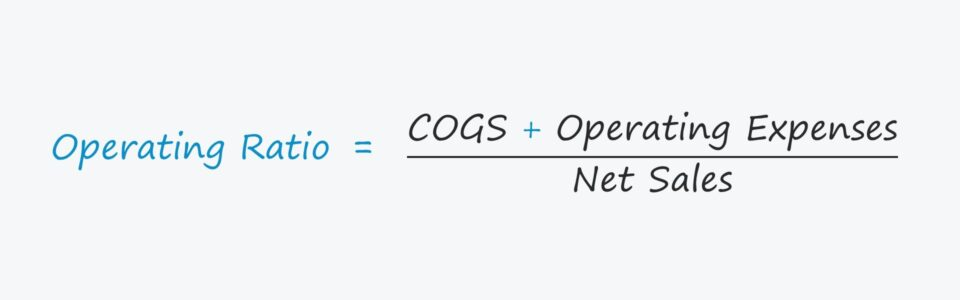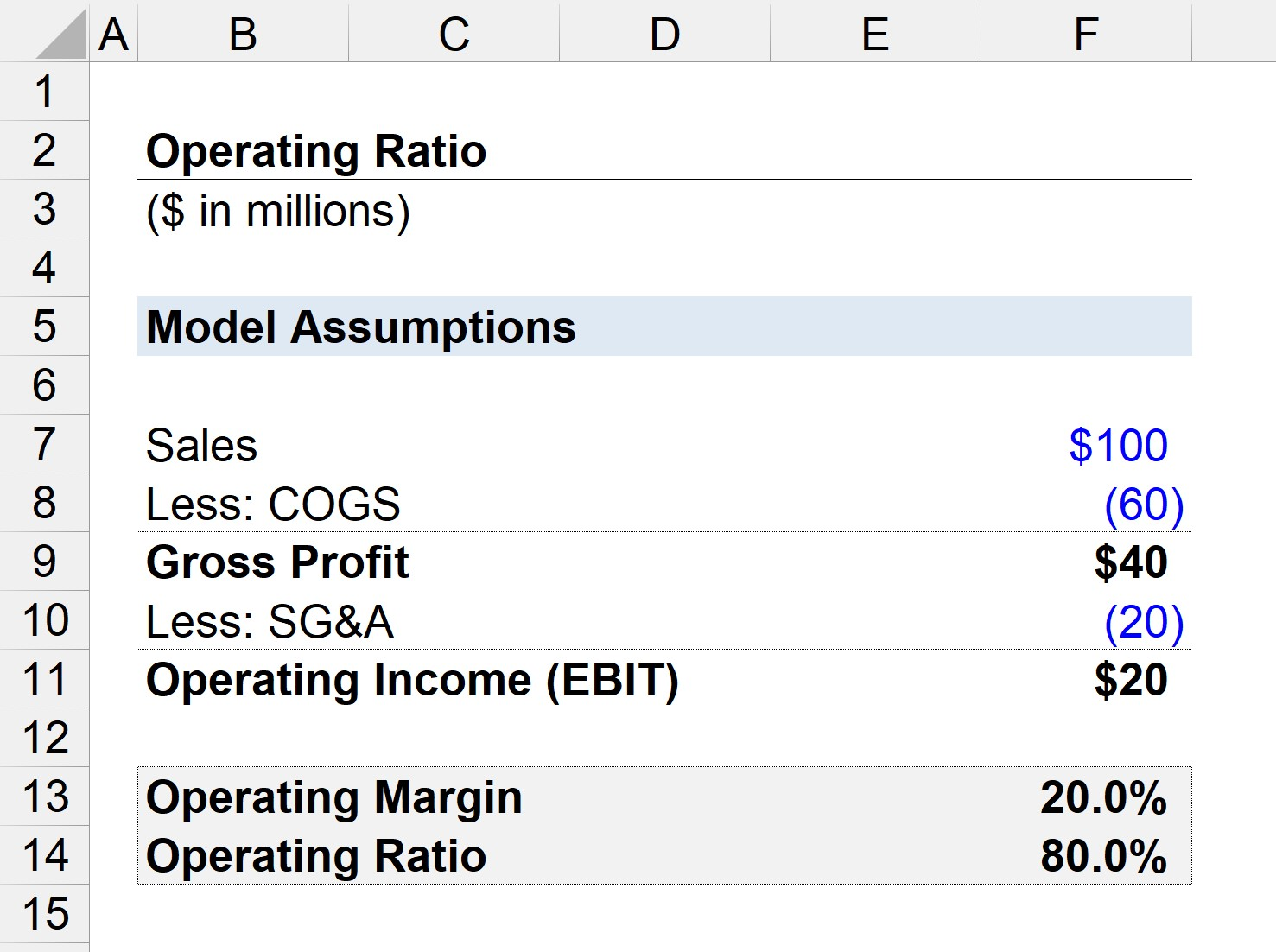Welcome to Wall Street Prep! Use code at checkout for 15% off.# Operating Ratio

Guide to Understanding the Operating Ratio Concept## How to Calculate Operating Ratio?

The operating ratio is calculated by dividing a company’s total operating costs by its net sales.

Sales represent the starting line item of the income statement (“top line”), whereas operating costs refer to the routine expenses incurred by a company as part of its normal course of operations.

Operating costs are comprised of two components: COGS and operating expenses:

1. Cost of Goods Sold (COGS): Otherwise known as the “cost of sales”, COGS represent the direct costs incurred by a company from selling its goods or services.
2. Operating Expenses (OpEx): Unlike cost of goods sold (COGS), operating expenses (or SG&A) are the costs not directly tied to how revenue is generated by a company, yet still have an integral role in its core operations.
Direct Operating Costs (COGS) Indirect Operating Costs (SG&A)
• Purchase of Inventory
• Research and Development (R&D)
• Cost of Raw Materials
• Sales and Marketing (S&M)
• Cost of Direct Labor
• Payroll and Wages

## Operating Ratio Formula

The formula for calculating the operating ratio divides a company’s operating costs by its net sales.

Operating Ratio = (COGS + Operating Expenses) / Net Sales

While a company’s sales can be easily found on the income statement, calculating a company’s total operating expenses requires adding up the appropriate expenses, as well as potentially removing the effects of certain non-recurring items.

If a company’s operating ratio is 0.60, or 60%, then this ratio means that \$0.60 is spent on operating expenses for each dollar of sales generated.

The remaining \$0.40 is either spent on non-operating expenses or flows down to net income, which can either be kept as retained earnings or issued as dividends to shareholders.

## What is a Good Operating Ratio?

In general, the lower the operating ratio, the more likely the company can efficiently generate profits.

One issue with the operating ratio is that the effects of operating leverage are neglected.

For instance, if a company with high operating leverage – i.e. more fixed costs than variable costs – is exhibiting strong growth in sales, the proportion of its total operating expenses relative to its sales tends to decline.

The company’s cost structure (and profit margins) are positioned to benefit from such cases, so the shift does not necessarily indicate that management is running the company any better.

Also, as with most ratios, comparisons with other companies are useful only if the chosen peer group consists of close competitors of a relatively similar size and maturity level.

When making historical comparisons with a company’s own year-over-year performance, the operating ratio can bring attention to the potential improvement in efficiency – but to reiterate from earlier, further investigation is required to determine the true cause of the improvement.

In other words, the operating ratio is most useful for preliminary analysis and spotting trends to further investigate, rather than as a standalone metric to directly reference and from which to make conclusions.

## Operating Ratio Calculator – Excel Template

We’ll now move to a modeling exercise, which you can access by filling out the form below.Submitting...

## Operating Ratio Calculation Example

Suppose we have a company that generated a total of \$100 million in sales, with \$50 million in COGS and \$20 million in SG&A.

• Sales = \$100 million
• COGS = \$60 million
• SG&A = \$20 million

After subtracting the company’s COGS from its net sales, we are left with \$40 million in gross profit (and 40% gross margin).

• Gross Profit = \$100 million – \$60 million = \$40 million
• Gross Profit Margin (%) = \$40 million / \$100 million = 40%

In the next step, we subtract SG&A – the only operating expense – from gross profit to calculate the company’s operating income (EBIT) of \$20 million (and 20% operating margin).

• Operating Income (EBIT) = \$40 million – \$20 million = \$20 million
• Operating Margin (%) = \$20 million / \$100 million = 20%

Using those assumptions, the total operating costs incurred by our company is \$80 million.

If we divide our company’s total costs by its net sales, the operating ratio comes out as 80% – which is the inverse of the 20% operating margin.

• Operating Ratio = (\$60 million + \$20 million) / \$100 million = 0.80, or 80%

The 80% operating ratio implies that if our company generates one dollar of sales, \$0.80 is spent on COGS and SG&A.Step-by-Step Online Course

### Learn Buy-Side (Hedge Fund) Modeling

Led by a former hedge fund PM (Maverick, Citadel, DE Shaw, Schonfeld), this program begins where financial modeling training ends — with a deep-dive into how buy-side analysts build financial models to make key investment decisions.